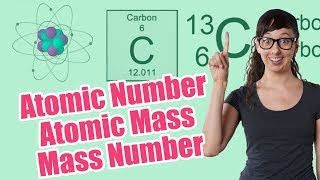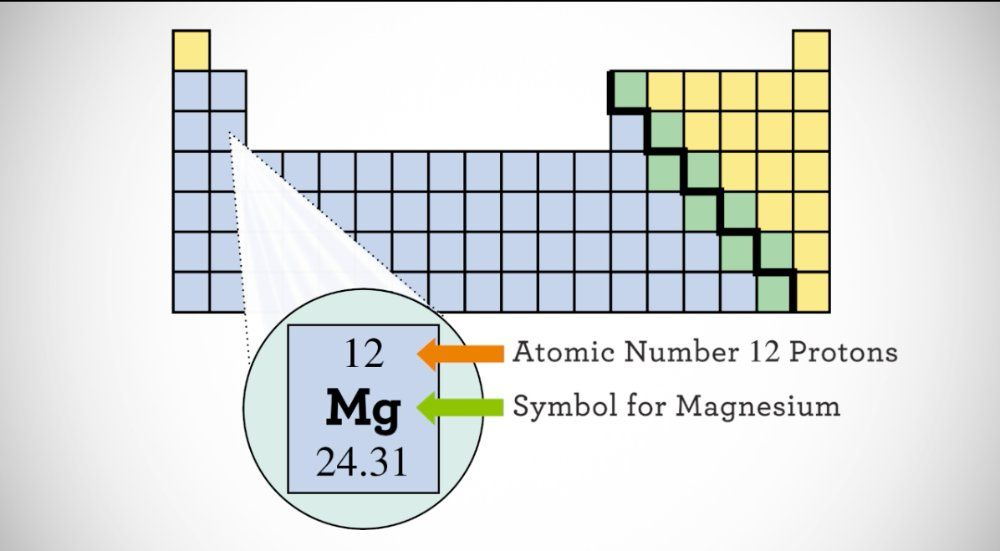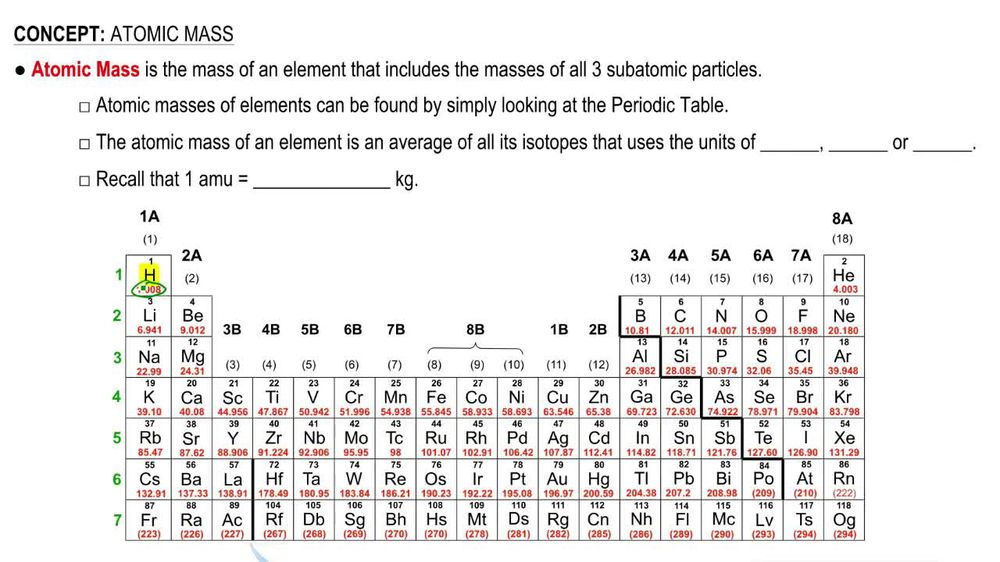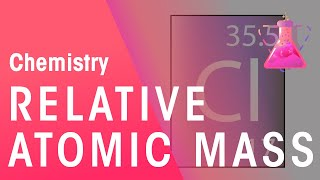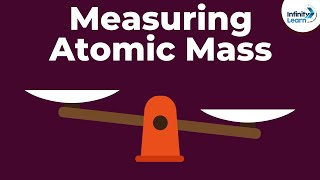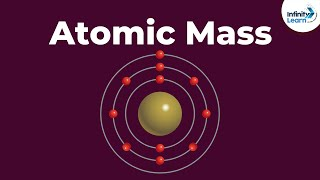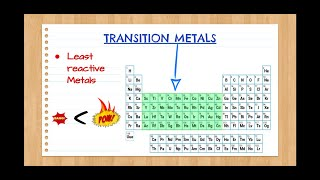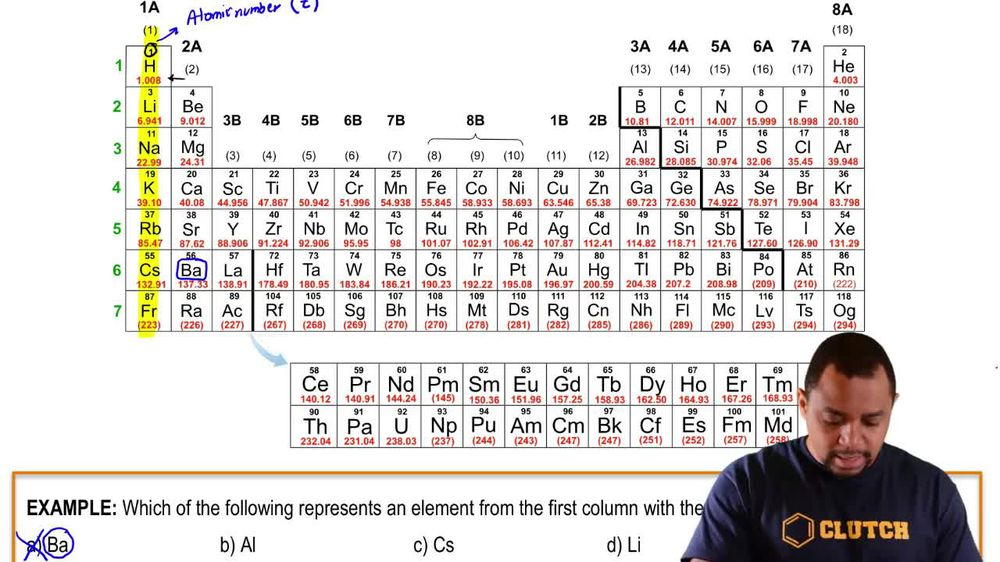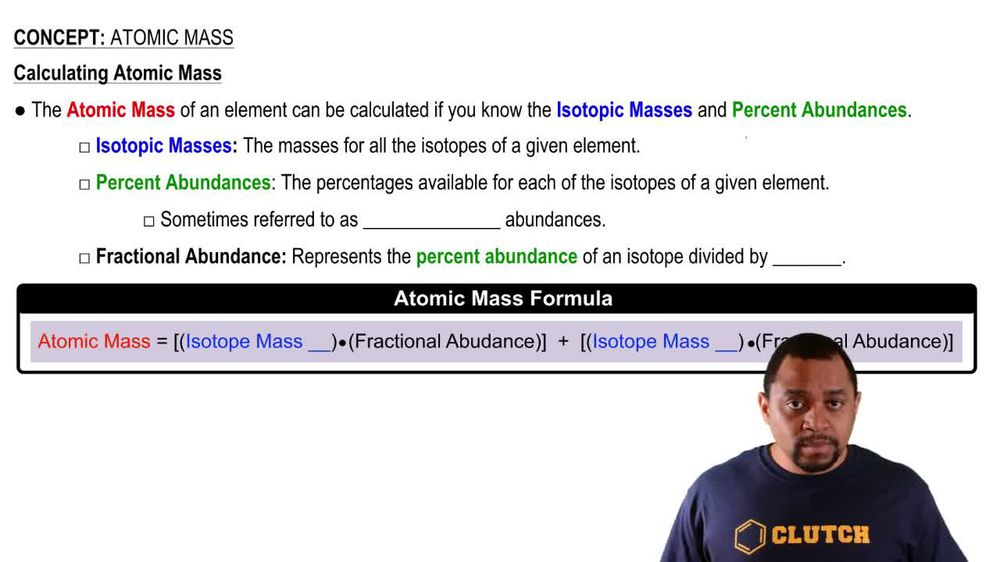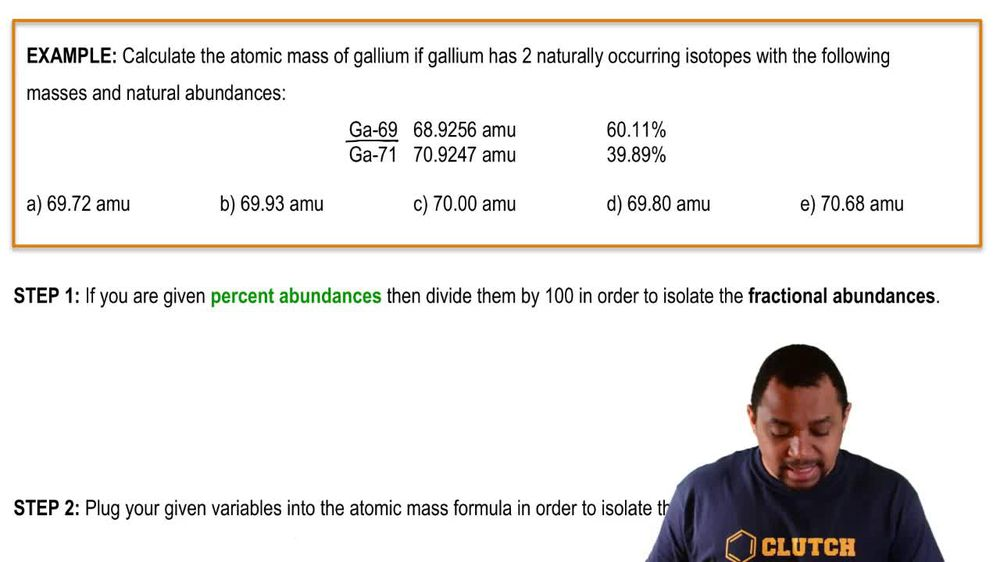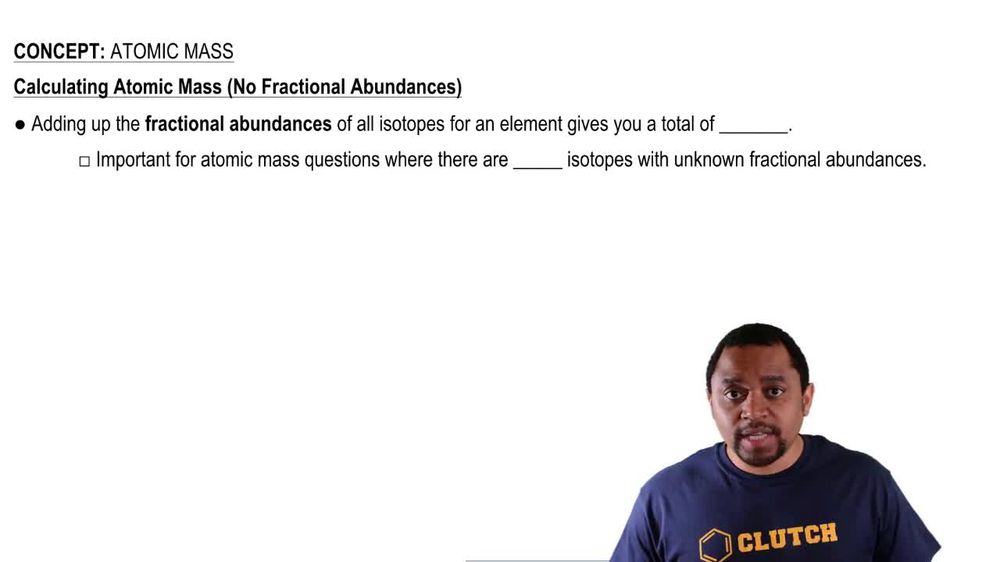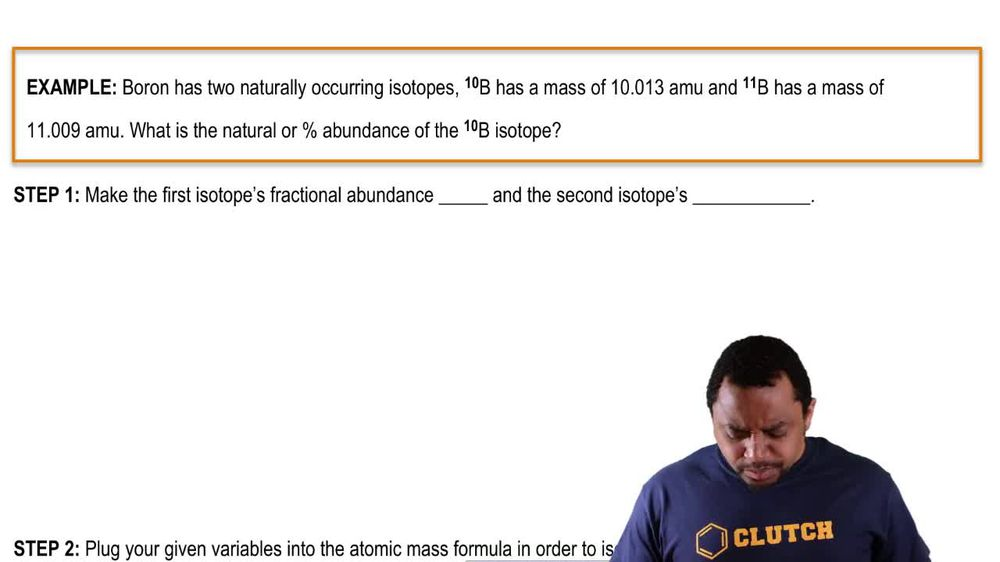Start typing, then use the up and down arrows to select an option from the list.
1. 2. Atoms & Elements2. Atomic Mass
Problem

# The element chromium (Cr) consists of four naturally occurring isotopes with atomic masses 49.9460, 51.9405, 52.9407, and 53.9389 u. The relative abundances of these four isotopes are 4.3, 83.8, 9.5, and 2.4%, respectively. From these data, calculate the atomic weight of chromium.

Relevant Solution3m
Play a video:
Hi everyone here we have a question telling us that lead has four stable isotopes listed with their atomic mass and abundance respectively. 204 lead 203.9730 Atomic mass units 1.4 206 lead to 5.9745 Atomic Mass units 24.1% 207 Lead, 206.9759 Atomic Mass units 22.1% and 208 lead 207.9767 Atomic Mass units 52.4%. And our goal is to calculate the atomic mass of blood. So to do that, we take our atomic mass units times are abundance for each isotope and add them together. So we're going to take 1.4 and it's a percent. So divided by Times .9730 Atomic mass units plus 24.1 Divided by Times .9745 Atomic mass units plus 22.1 divided by Times 206.9759 Atomic mass units plus 52.4, Divided by Times 207.9767 Atomic Mass Units. And that equals 2. plus 49. plus 45. Plus 108.9798 And that equals 207. Atomic mass units. And that is our final answer. Thank you for watching Bye.